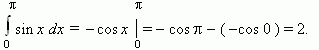# Definite integral. Newton – Leibniz formula

Curvilinear trapezoid. Area of a curvilinear trapezoid.
Definite integral. Limits of integration. Integrand.
Newton-Leibniz formula.

Consider a continuous function y = f ( x ), given on a segment [ a , b ] and saving its sign on this segment ( Fig.8 ). The figure, bounded by a graph of this function, a segment [ a , b ] and straight lines x = a and x = b , is called a curvilinear trapezoid . To calculate areas of curvilinear trapezoids the following theorem is used:

If  f  – a continuous, non-negative function on a segment [

a , b ], and  F – its primitive on this segment, then an area  S  of the corresponding curvilinear trapezoid is equal to an increment of the primitive on a segment [ a, b ], i.e.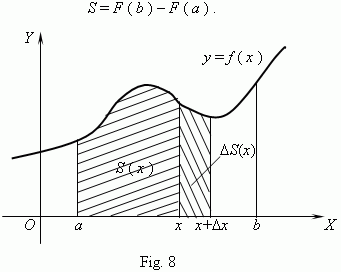Consider a function S ( x ), given on a segment [ a , b ]. If a < x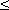b ,  then S ( x ) is an area of the  part of the curvilinear trapezoid, which is placed on the left of a vertical straight line, going through the point ( x , 0 ). Note, that if x = a , then S ( a ) = 0  and S ( b ) = S ( S – area of the curvilinear trapezoid ). It is possible to prove, that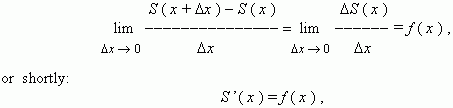i.e. S ( x ) is a primitive for f ( x ). Hence, according to the basic property of primitives, for all x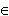[ a , b ]  we have:

S ( x ) = F ( x ) + C ,

where C – some constant, F – one of the primitives for a function f .
To find C we substitute x = a :

F ( a ) + C = S ( a ) = 0,

hence, C = - F ( a ) and S ( x ) = F ( x ) - F ( a ). As an area of the curvilinear trapezoid is equal to S ( b ) , substituting x = b , we’ll receive:

S = S ( b ) = F ( b ) - F ( a ).

E x a m p l e .  Find an area of a figure, bounded by  the curve y = x 2 and lines
y = 0, x = 1, x = 2  ( Fig.9 ) .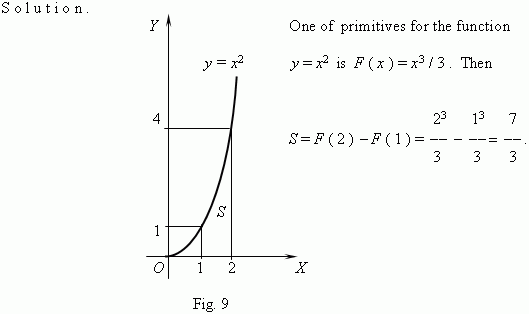Definite integral. Consider another way to calculate an area of a curvilinear trapezoid. Divide a segment [ a , b ] into n segments of an equal length by points:

x 0 = a < x 1 < x 2 < x 3 < …< x n - 1 < x n = b

and let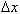= ( b a ) / n = x k - x k - 1 ,  where k = 1,  2, …, n – 1, n . In each of  segments [ x k - 1 , x k ]  as on a base we’ll build a rectangle of  height f ( x k - 1 ) . An area of this rectangle is equal to: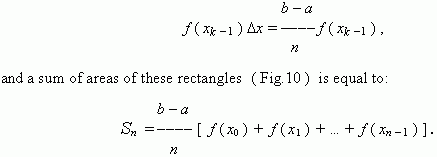In view of continuity of a function f ( x )  a union of the built rectangles at great n (i.e. at small) "almost coincides" with our curvilinear trapezoid. Therefore, S n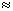S at great values of n . It means, that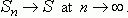This limit is called an integral of a function f ( x ) from a to b or a definite integral :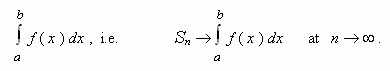Numbers a and b are called limits of integration , f ( x ) dx – an integrand . So, if f ( x )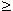0 on a segment [ a , b ] , then an area S of the corresponding curvilinear trapezoid is represented by the formula: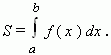Newton Leibniz formula. Comparing the two formulas of the curvilinear trapezoid area, we make the conclusion: if F ( x ) is primitive for the function f ( x )  on a segment  [ a , b ] , then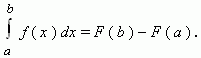This is the famous Newton – Leibniz  formula. It is valid for any function f ( x ), which is continuous on a segment  [ a , b ] .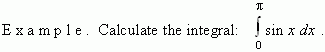S o l u t i o n.   Using the table of integrals for some elementary functions ( see above ), we’ll receive: